# DC Pandey Solutions: Calorimetry & Heat Transfer Notes | Study Physics Class 11 - NEET

## NEET: DC Pandey Solutions: Calorimetry & Heat Transfer Notes | Study Physics Class 11 - NEET

The document DC Pandey Solutions: Calorimetry & Heat Transfer Notes | Study Physics Class 11 - NEET is a part of the NEET Course Physics Class 11.
All you need of NEET at this link: NEET

Introductory Exercise 19.1

Take cice = 0.53 cal/g-°C, cwater = 1.0 cal/g-°C,(Lf)water = 80cal/g and(Lv)water = 529 cal/g unless given in the question,
Ques 1: In a container of negligible mass 140 g of ice initially at -15° C is added to 200 g of water that has a temperature of 40°C. If no heat is lost to the surroundings, what is the final temperature of the system and masses of water and ice in mixture?
Sol: Let mixture is water at θ°C (where 0°C< θ < 40°C)
Heat given by water = Heat taken by ice
∴  (200) (1) (40 - θ) = (140) (0.53) (15) + (140)(80)+(140)(l)(θ - 0)
Solving we get,
θ = -12.7°C
Since, θ < 0° C and we have assumed the mixture to be water whose temperature can't be less than 0°C.
Hence, mixture temperature θ = 0° C. Heat given by water in reaching upto 0° C is,
θ = (200) (1) (40 - 0) = 8000 cal.
Let m mass of ice melts by this heat, then 8000 = (140) (0.53) (15)+(m) (80)
Solving we get m = 86 g
∴  Mass of water = 200 + 86 = 286 g
Mass of ice = 140 - 86 = 54 g

Ques 2: The temperatures of equal masses of three different liquids A, Sand Care 12°C, 19°C and 28°C respectively. The temperature when A and B are mixed is 16°C and when S and C are mixed is 23°C. What would be the temperature when A and C are mixed?
Sol:
A + B msA (16 - 12) = msB (19' - 16)
4sA = 3sB
B + C msB (23 - 19) = msC (28 - 23)
4s= 5sC ...(ii)
Solving these two equations, we get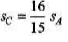A + C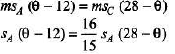Solving, we get
θ = 20.25° C

Ques 3: Equal masses of ice (at 0°C) and water are in contact. Find the temperature of water needed to just melt the complete ice.
Sol:
mL = ms (θ - 0°)
∴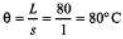Ques 4: A nuclear power plant generates 500 MW of waste heat that must be carried away by water pumped from a lake. If the water temperature is to rise by 10°C, what is the required flow rate in kg/s?
Sol: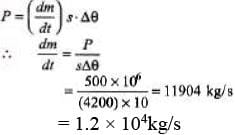Introductory Exercise 19.2

Ques 1: Suppose a liquid in a container is heated at the top rather than at the bottom. What is the main process by which the rest of the liquid becomes hot?
Sol:
In convection, liquid is heated from the bottom.

Ques 2: The inner and outer surfaces of a hollow spherical shell o f inner radius 'a' and outer radius 'b' are maintained at temperatures T1 and T2 (< T1). The thermal conductivity of material of the shell is k. Find the rate of heat flow from inner to outer surface.
Sol:
Let us take an element at distance r from centre of thickness dr. Applying the formula of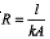or thermal resistance.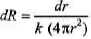= thermal resistance of this element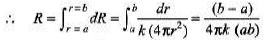Rate of heat flow,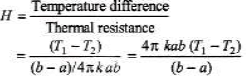Ques 3: Show that the SI units of thermal conductivity are W/m-K.
Sol: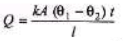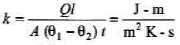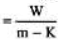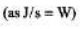Ques 4: A carpenter builds an outer house wall wit h a layer of wood 2.0 cm thick on the outside and a layer of an insulation 3.5 cm thick as the inside wall surface. The wood has k = 0.08 W/m -K and the insulation has k = 0.01 W/m -K. The interior surface temperature is 19° C and the exterior surface temperature is -10° C.
Sol:
(a) H1 = H2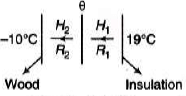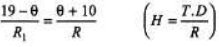or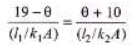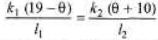∴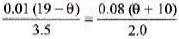Solving, we get
θ= -8.1°C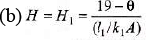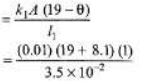= 7.7 W/m2

Ques 5: A pot with a steel bottom 1.2 cm thick rests on a hot stove. The area of the bottom of the pot is 0.150 m2. The water inside the pot is at 100°C and 0.440 kg are evaporated every 5.0 minute. Find the temperature of the lower surface of the pot, which is in contact with the stove. Take Lv = 2.256 × 10J/kg and ksteel = 50.2 W/m-K.
Sol: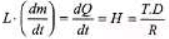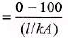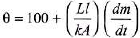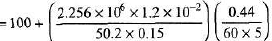= 105°C

Ques 6: A layer of ice o f thickness y is on the surface of a lake. The air is at a constant temperature -θ°C and the ice water interface is at 0°C. Show that the rate at which the thickness increases is given by,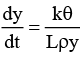where k is the thermal conductivity of the ice, L the latent heat of fusion and p is the density of the ice.
Sol:
See the extra points just before solved examples. Growth of ice on ponds. We have already derived that,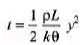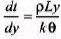∴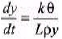Ques 7: The emissivity of tungsten is 0.4. A tungsten sphere with a radius of 4.0 cm is suspended within a large evacuated enclosure whose walls are at 300 K. What power input is required to maintain the sphere at a temperature of 3000 K if heat conduction along supports is neglected?
Take σ= 5.67 × 10-8 W/m2 -K4.
Sol: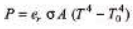= (0.4)(5.67 × 10-8)(4π)(4 × 10-2)2[3000)4 -(300)4]
= 3.7 × 104W

Ques 8: Find SI units of thermal resistance.
Sol: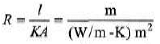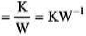The document DC Pandey Solutions: Calorimetry & Heat Transfer Notes | Study Physics Class 11 - NEET is a part of the NEET Course Physics Class 11.
All you need of NEET at this link: NEETUse Code STAYHOME200 and get INR 200 additional OFF

## Physics Class 11

80 videos|393 docs|210 tests

### How to Prepare for NEET

Read our guide to prepare for NEET which is created by Toppers & the best Teachers

Track your progress, build streaks, highlight & save important lessons and more!

,

,

,

,

,

,

,

,

,

,

,

,

,

,

,

,

,

,

,

,

,

;